# 基于SVM-KNN的股票价格预测Stock Price Forecast Based on SVM-KNN

DOI: 10.12677/SA.2019.86097, PDF, HTML, XML, 下载: 345  浏览: 2,008

Abstract: In this paper, the support vector machine (SVM) and K-nearest neighbor (KNN) algorithm were used to study the stock price forecasting problem, select the transaction data reflecting the stock changes and its technical indicators, including volume, closing price, highest price, moving average (MA), etc., forecasting the ups and downs and the closing price of the Shanghai Composite Index. Firstly, the SVM was used to predict the training set’s ups and downs. Then the training set used KNN to predict the short-term (1 day), medium-term (7 days) and long-term (30 days) prices of the stock, thus forming a forecast model based on transaction data and technical indicators. Finally, the MAPE and RMSE of this model were obtained. In order to verify the validity of the model, a new investment strategy was constructed based on the model prediction results, and the real data was used for investment, and the large-cap stock index was subjected to a one-month simulation investment.

1. 引言

2. 数据与指标

2.1. 数据来源

2.2. 数据整理

$U=\left[\begin{array}{ccccccc}{u}_{1p}& {u}_{1o}& {u}_{1h}& {u}_{1l}& {u}_{1c}& {u}_{1n}& {u}_{1v}\\ {u}_{2p}& {u}_{2o}& {u}_{2h}& {u}_{2l}& {u}_{2c}& {u}_{2n}& {u}_{2v}\\ {u}_{3p}& {u}_{3o}& {u}_{3h}& {u}_{3l}& {u}_{3c}& {u}_{3n}& {u}_{3v}\\ ⋮& ⋮& ⋮& ⋮& ⋮& ⋮& ⋮\\ {u}_{np}& {u}_{no}& {u}_{nh}& {u}_{nl}& {u}_{nc}& {u}_{nn}& {u}_{nv}\end{array}\right]$ (1)

$\Delta {c}^{i}={u}_{i+m,c}-{u}_{i,c},\forall i=1,2,3,\cdots ,n-1$ (2)

${y}_{i}=\left\{\begin{array}{l}-1,\text{\hspace{0.17em}}\text{\hspace{0.17em}}\Delta {c}^{i}<0\\ 1,\text{\hspace{0.17em}}\text{\hspace{0.17em}}\text{\hspace{0.17em}}\text{\hspace{0.17em}}\Delta {c}^{i}\ge 0\end{array}$ (3)

${u}_{ij}=\frac{{u}_{ij}-{u}_{\mathrm{min}j}}{{u}_{\mathrm{max}j}-{u}_{\mathrm{min}j}}$ (4)

2.3. 技术分析指标

HSU  指出，在机器学习以前，金融经济学家一般更喜欢使用计量经济学，选择技术指标利用EMH模型来对股市进行分析。而技术指标依赖于过去的价格和成交量确定未来的价格趋势。现有的研究一般是基于多种技术指标构建交易策略提高盈利能力，包括过滤器规则  、移动平均线  、动量  和自动模式识别 。因此，本文在收集到的原始数据的基础上加入一些重要的技术指标构成最终的特征向量。加入的技术指标如表一所示：

$U=\left[\begin{array}{ccccccccccccc}{u}_{1p}& {u}_{1o}& {u}_{1h}& {u}_{1l}& {u}_{1c}& {u}_{1n}& {u}_{1v}& {T}_{11}& {T}_{12}& {T}_{13}& {T}_{14}& {T}_{15}& {c}_{1}\\ {u}_{2p}& {u}_{2o}& {u}_{2h}& {u}_{2l}& {u}_{2c}& {u}_{2n}& {u}_{2v}& {T}_{21}& {T}_{22}& {T}_{23}& {T}_{24}& {T}_{25}& {c}_{2}\\ {u}_{3p}& {u}_{3o}& {u}_{3h}& {u}_{3l}& {u}_{3c}& {u}_{3n}& {u}_{3v}& {T}_{31}& {T}_{32}& {T}_{33}& {T}_{34}& {T}_{35}& {c}_{3}\\ ⋮& ⋮& ⋮& ⋮& ⋮& ⋮& ⋮& ⋮& ⋮& ⋮& ⋮& ⋮& ⋮\\ {u}_{np}& {u}_{no}& {u}_{nh}& {u}_{nl}& {u}_{nc}& {u}_{nn}& {u}_{nv}& {T}_{n1}& {T}_{n2}& {T}_{n3}& {T}_{n4}& {T}_{n5}& {c}_{n}\end{array}\right]$ (5)

2.4. 误差分析

$\text{MAPE}=\frac{1}{N}{\sum }_{i=1}^{N}|\frac{{u}_{i,\text{close}}-{\stackrel{^}{u}}_{i,\text{close}}}{{u}_{i,\text{close}}}|\ast 100$ (6)

$\text{RMSE}=\sqrt{\frac{1}{N}\underset{i=1}{\overset{N}{\sum }}{\left({u}_{i,\text{close}}-{\stackrel{^}{u}}_{i,\text{close}}\right)}^{2}}$ (7)

3. 基于SVM-KNN的预测模型

3.1. 支持向量机分类算法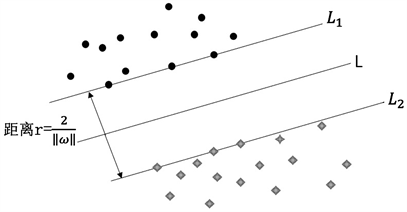Figure 1. Linear plane separable hyperplane

$\mathrm{min}\Phi \left(x\right)=\frac{{‖w‖}^{2}}{2}+C{\sum }_{i=1}^{n}{\xi }_{i}$ (7)

s.t $\left\{\begin{array}{l}{y}_{i}\left({w}^{\text{T}}{x}_{i}+b\right)\ge 1-{\xi }_{i}\\ {\xi }_{i}\ge 0,\text{\hspace{0.17em}}i=1,2,3,\cdots ,n\end{array}$

$\mathrm{max}Q\left(\alpha \right)={\sum }_{i=1}^{n}{\alpha }_{i}-\frac{1}{2}{\sum }_{i=1}^{n}{\sum }_{j=1}^{n}{\alpha }_{i}{\alpha }_{j}{y}_{i}{y}_{j}〈\varphi \left({x}_{i}\right),\varphi \left({x}_{j}\right)〉$ (8)

s.t $\left\{\begin{array}{l}{\sum }_{i=1}^{n}{\alpha }_{i}{y}_{i}=0\\ 0\le {\alpha }_{i}\le C,i=1,2,3,\cdots ,n\end{array}$

$f\left(x\right)=\mathrm{sgn}\left\{\left({w}^{\text{T}}\cdot \varphi \left(x\right)\right)\right\}+b=\mathrm{sgn}\left\{{\sum }_{支持向量}{\alpha }_{i}^{*}{y}_{i}〈\varphi \left({x}_{i}\right),\varphi \left(x\right)〉+{b}^{*}\right\}$ (9)

$K\left({x}_{i},{x}_{j}\right)=\mathrm{exp}\left(-\gamma {‖{x}_{i}-{x}_{j}‖}^{2}\right),\gamma >0$ (10)

3.2. K近邻分类算法

KNN (K-Nearest-Neighbor)分类器是通过给定一个待识别的样本集，根据在训练集中寻找到最近的K个近邻，通过确定K的个数，从而将待识别样本进行归类。图2中给出的是在k = 5的条件下的分类状况。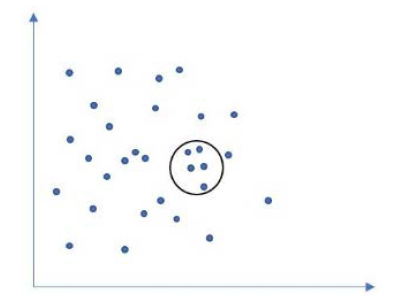Figure 2. Classification when K = 5

3.3. SVM-KNN算法实现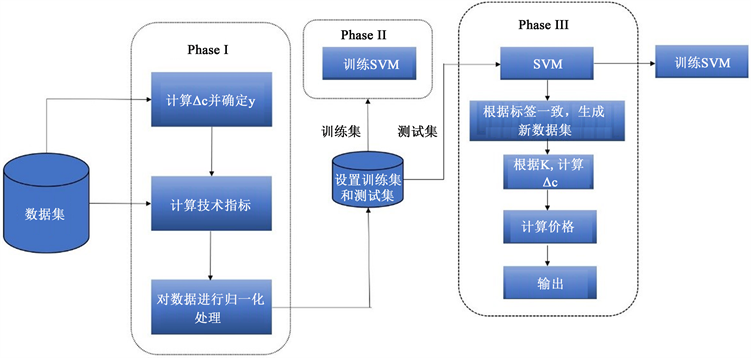Figure 3. Algorithm flowchartTable 1. Technical index calculation formula

4. 实例验证与结果分析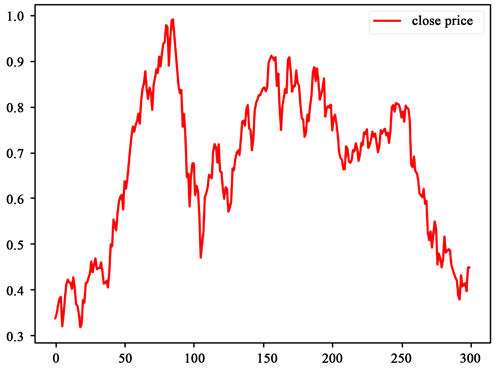Figure 4. Normalized closing price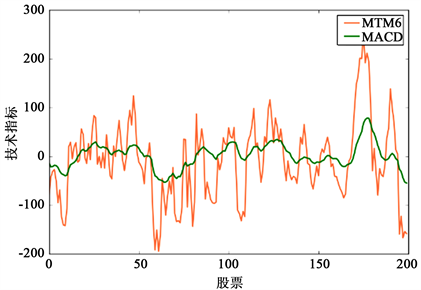Figure 5. MTM and MACD indicators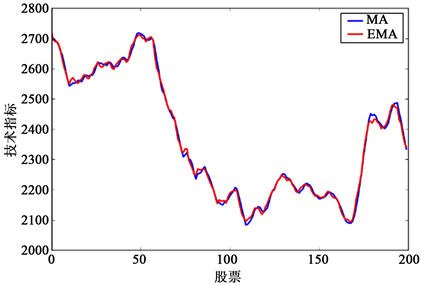Figure 6. MA and EMA indicators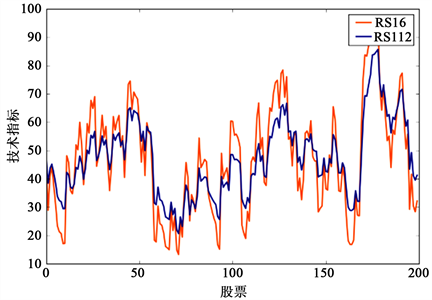Figure 7. RSI indicatorsTable 2. Parameter selection of SVMTable 3. SVM’s forecast of the ups and downs of the Shanghai Composite Index on different periodsTable 4. MAPE and RMSE calculation errors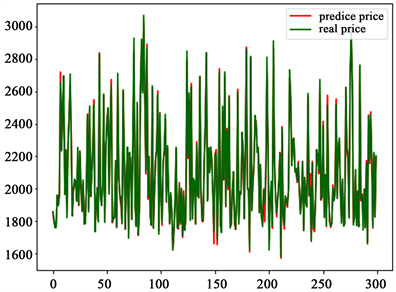Figure 8. One-day ups and downs forecast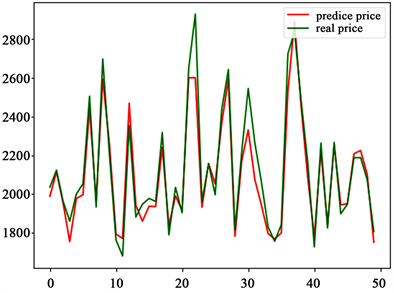Figure 9. Seven-day ups and downs forecast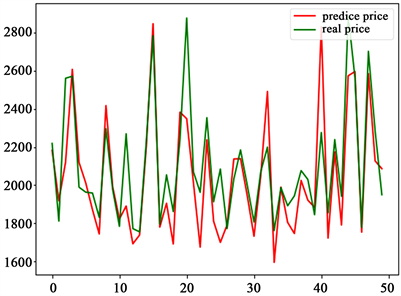Figure 10. Thirty-day ups and downs forecast

5. 模拟投资

5.1. 构建投资策略Table 5. Thirty-day rise and fall

5.2. 计算收益

t日实施投资并持有两天以上后将获得的股票当日收益率为：

${R}_{t}=\frac{{u}_{t+n}-{u}_{t}}{{u}_{t}}$ (11)

$\text{CumR}=\underset{t=1}{\overset{n}{\prod }}\left(1+{R}_{t}\right)-1$ (12)Figure 11. Thirty-day closing price

5.3. 结果分析

$\text{Sharperatio}=\frac{E\left({R}_{p}\right)-{R}_{f}}{{\sigma }_{P}}$ (13)

6. 总结